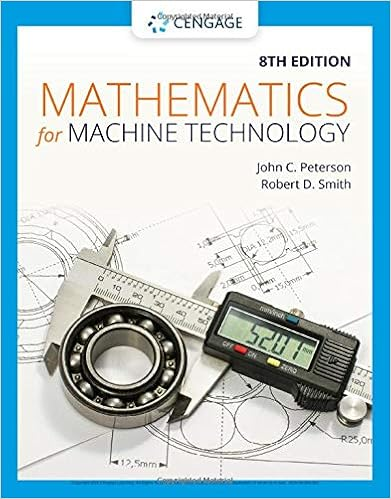# Physics 102 Exam 1 - Test#1 Physics 102 Answer all problems...

• Test Prep
• 5
• 75% (12) 9 out of 12 people found this document helpful

This preview shows page 1 - 2 out of 5 pages.

##### We have textbook solutions for you!
The document you are viewing contains questions related to this textbook.The document you are viewing contains questions related to this textbook.
Chapter 5 / Exercise 13b
Mathematics for Machine Technology
Peterson/SmithExpert Verified
/ Physics 102 Test #1 February 10, 2014 Answer all problems. Clearly illustrate the basic principles and show all steps. From your syllabus: Full credit for exam problems is only possible when the steps used to reach the solution, actual insertion of any numbers and clear identification of the answer are done. Circle your section: -8:30 AM or (0 :30 AM ~ Last Name ---- ~ ~ ~ ~~ ----------------- First Name - (!{{~ ---------------- v=vo+at F=qE P=IAV 1 ~? 2 i'? 3 /_!?_ 4- ~ s- :t 6 _] - ~ 1-- '- s L'? Extra£ TotalL 03 [email protected] )( \Ob d= V 0 t + Y2 ar I= AQ/At v 2 =v 0 2 + 2ad KE = Yz mv 2 F =rna a=v 2 /r F = kqQ/~ V = kQ/r AV = APE/q AV= -Ed I= A VIR P = energy/t energy =112 C AV 2 R= p L/A C = Q/AV C = £,A./d e =1 6xl0' 19 C k= 9xl0 9 Nm 2 /C 2 , E: 0 = 8.85xl0' 12 C 2 /N.m 2 , Prot~n mass =\ .67xl0- 27 kg, speed of light = 3xl0 8 m/s, Electron mass= 9.lxi0- 31 kg, speed of sound = 343 m/s. 1. Problem potpourri: ~ 1. (2 pts) Does the capacitor stores o~ds electric energy? s-h>rt.~ 11 . (5 pts) F' d the electric force between Na + and Cl' in salt? The distance between the two ions is x 10 ' 10 m? - l<~ll _,,. 1 ~ l ~t-l~t,llct :LI ::- ( ~) .. 11 ·~~ 113 -l.btloJ ~ fJ Gil X IO-Cf .I F ~ r'l.. qll\0 (~.gllllo-lu)1 0• - lc;< N \...---------' 111. ( 4 pts) An atom has three electrons, three protons and three neutrons. A) What is the net charge of the atom? 0' l~) . B)'What is the net charge of the atom when it loses one electron? t»~iHvc. C+-) 'rt ole C) What is the net charge of the atom when it gains one electron? ~ c. -\-i v~ t-) TV\~ J 1\, V · D) What is the net charge of the atom when it loses one proton?
##### We have textbook solutions for you!
The document you are viewing contains questions related to this textbook.The document you are viewing contains questions related to this textbook.
Chapter 5 / Exercise 13b
Mathematics for Machine Technology
Peterson/SmithExpert Verified
•••